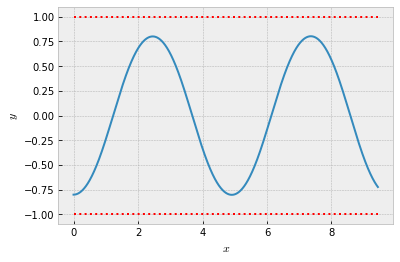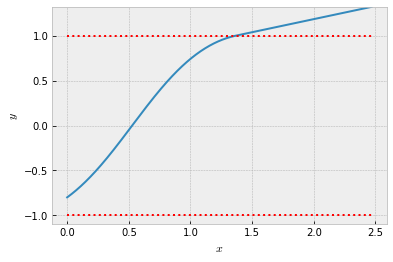# Diffraction in a Gradient-index Optical Fiber¶

### Examples - Astrophysics¶

Last edited: March 22nd 2018

### Snell's Law - The Law of Refraction¶

Snell’s Law describes what happens to light as it enters one medium from another. More specifically, it relates the direction of the light in each medium to the velocity of the light in each medium.

Equivalently, it relates the angles of incident, $\theta$, to the index of refraction, $n$, of each medium (see figure below). In its most common form, Snell’s law reads $$n_1 \sin\theta_1 = n_2 \sin\theta_2,$$ where the index of refraction can be defined as $$n_i = \frac{c}{v_i}.$$ Here, $v_i$ is the speed of light in a specific medium and $c$ is the speed of light in vacuum. Snell’s Law can be derived in multiple ways. One method is to use the so-called Fermat’s Principle of Least Time.In the figure $n_1<n_2$, and hence $\theta_1>\theta_2$. For instance, this is the case for air ($n_\mathrm{air} \approx 1.0003$) and water ($n_\mathrm{water} \approx 1.333$).

### Fermat's Principle of Least Time¶

Fermat’s Principle states that light follows the path of least time when moving from point A to point B. If the speed of light is constant - or equivalently, if the index of refraction is constant - then light follows straight lines. The distance, $s$, travelled by the light during time $t$ is then $$s = vt \Leftrightarrow t = \frac{s}{v}.$$ ￼If the index of refraction is not constant, the light path is not a straight line anymore. Instead, we encounter diffraction.

In Newtonian mechanics, the equation of motion in two dimensions corresponding to a straight line, is simply that of zero acceleration: $$\frac{d^2x}{dt^2}=0,\quad \frac{d^2y}{dt^2} =0.$$ We will now investigate if there is an equivalent equation for light rays if the index of refraction varies continuously in a medium, instead of discretely as in the case of Snell’s Law.

### Diffraction¶

To apply Fermat’s Principle, we need an expression for the time, $T$ , it takes light to go from a point A to a point B. In a small amount of time, $dt$, light travels a distance $ds = v \cdot dt$. If the index of refraction is continuous and differentiable (smooth), then $v$ is approximately constant in a sufficiently small time interval.

Summing up all the time intervals along the path gives the total time $$T = \int_A^B dt = \int_A^B \frac{ds}{v} = \frac{1}{c} \int_A^B n\,ds.$$ The last integral is called the optical path length. Therefore, finding the minimum time is equivalent to finding the minimum optical path length. Solving this problem is not trivial and we only sketch the solution here.

If we minimize the last integral, we will find that this leads to the following Euler-Lagrange equations for the motion of light where the index of refraction is of the form $n = n(x,y)$ (i.e. diffraction): \begin{align} \frac{d^2x}{d\eta^2} &= \frac{1}{n}\left[-\frac{\partial n}{\partial x}\left(\frac{dx}{d\eta}\right)^2-2\frac{\partial n}{\partial y}\left(\frac{dx}{d\eta}\right)\left(\frac{dy}{d\eta}\right)+\frac{\partial n}{\partial x}\left(\frac{dy}{d\eta}\right)^2\right]\\ \frac{d^2y}{d\eta^2} &= \frac{1}{n}\left[\frac{\partial n}{\partial y}\left(\frac{dx}{d\eta}\right)^2-2\frac{\partial n}{\partial x}\left(\frac{dx}{d\eta}\right)\left(\frac{dy}{d\eta}\right)-\frac{\partial n}{\partial y}\left(\frac{dy}{d\eta}\right)^2\right]. \end{align} Here, $\eta$ parametrizes the path since the right-hand side does not contain $c$ or anything else from which to obtain a time scale. Note that if $n$ is constant, $$\frac{\partial n}{\partial x} = 0 \text{ and } \frac{\partial n}{\partial y} = 0$$ hold, and we simply recover the equations for a straight line. Now, we only need to solve these two coupled, nonlinear differential equations to obtain the path of light.

### Numerical Solution¶

Solving the previous two equations analytically is no easy task and is impossible in most cases. Solving them numerically, on the other hand, is almost trivial. We will use Euler integration.

Defining $$v_x = \dot{x} = \frac{dx}{d\eta},\, v_y = \dot{y}=\frac{dy}{d\eta},\, n_x = \frac{\partial n}{\partial x} \text{ and } n_y = \frac{\partial n}{\partial y},$$ we transform the two coupled second-order differential equations into four first-order differential equations: \begin{align} \dot{x} &= v_x,\\ \dot{y} &= v_y,\\ \dot{v}_x &= -\frac{n_x}{n}v_x^2-2\frac{n_y}{n}v_x v_y + \frac{n_x}{n}v_y^2,\\ \dot{v}_y &= \frac{n_y}{n}v_x^2-2\frac{n_x}{n}v_x v_y + \frac{n_y}{n}v_y^2. \end{align}

The corresponding recursive formulas for the position and velocity follow from Euler’s method, i.e. from discretizing the derivatives: \begin{align} x(\eta + \Delta \eta) &= x(\eta) + v_x(\eta)\Delta \eta,\\ y(\eta + \Delta \eta) &= y(\eta) + v_y(\eta)\Delta \eta,\\ v_x(\eta + \Delta \eta) &= v_x(\eta) + \dot{v}_x(\eta)\Delta \eta,\\ v_y(\eta + \Delta \eta) &= v_y(\eta) + \dot{v}_y(\eta)\Delta \eta.\\ \end{align}

### Example: Gradient-index (GRIN) Optical Fiber¶

A gradient-index optical fiber is a cylindrical (circular) cable with an index of refraction that varies with the distance from the center. To simplify matters, we will only simulate this in two-dimensional Cartesian coordinates $(x,y)$. Let the index of refraction be given by the function $n(x, y) = 2 − y^2$ for $|y| < 1$ and $n(x,y) = 1$ otherwise. This corresponds to a fiber cable of width 2 along the $x$-axis, surrounded by air or vacuum. The partial derivatives are $n_x = 0$ and $n_y = −2y$ inside the cable, and zero outside. From this, we get \begin{align} \dot{v}_x &= \frac{4y}{2-y^2}v_x v_y,\\ \dot{v}_y &= \frac{2y}{2-y^2}v_y^2 - \frac{2y}{2-y^2}v_x^2. \end{align}

We have implemented this example below. It shows the working principle of such optical fibers, although we demonstrate it only in two dimensions. Three dimensions would require cylindrical coordinates but the principle is the same.

Note that we have chosen $t$ instead of $\eta$ below to simplify notation.

In :
import numpy as np
from math import atan2
import matplotlib.pyplot as plt
plt.style.use('bmh')

In :
def lightray(x0, y0, angle, tmax, dt):
""" Returns n0*sin(theta0) as the first output, and n*sin(theta)
as the last one. n0 is the index of refraction at (x0,y0)
while n is the index of refraction at (x(tmax),y(tmax)).
theta0 is the initial angle with respect to the y-axis, and
theta is the angle with respect to the y-axis at the last time step.

Veryfying Snell's law:
n1*sin(t1) = n2*sin(t2)
"""
nmax = int(tmax/dt)
t = np.linspace(0, tmax, nmax)

x = 0*t
y = 0*t
vx = 0*t
vy = 0*t

x = x0
y = y0
vx = np.sin(np.pi*angle/180)/(2-y**2)   #Normalized, v = c/n
vy = np.cos(np.pi*angle/180)/(2-y**2)   #Normalized, v = c/n

for n in range(nmax-1):
x[n+1] = x[n] +  vx[n]*dt
y[n+1] = y[n] + vy[n]*dt
if abs(y[n]) <= 1:
factor = 2*y[n]/(2-y[n]**2)
vx[n+1] = vx[n] + 2*factor*vx[n]*vy[n]*dt
vy[n+1] = vy[n] +   factor*(vy[n]**2 - vx[n]**2)*dt
else:
vx[n+1] = vx[n]
vy[n+1] = vy[n]

plt.plot(x,y,[x,x[-1]],[1,1],':r',[x,x[-1]],[-1,-1],':r')
plt.ylim([min(min(y),-1.1), max(max(y),1.1)])
plt.xlabel(r"$x$")
plt.ylabel(r"$y$")

# Verifying Snell's Law:
# The angle is measured with respect to the x-axis. Rotates the angle
# by 90 degrees to be with respect to the y-axis.
aa = atan2(vx[-1],vy[-1])*180/np.pi
if abs(y[-1]) < 1:
nn = 2 - y[-1]**2
else:
nn = 1

an1 = (2-y0**2)*np.sin(angle*np.pi/180)  #Initial result
an2 = nn*np.sin(aa*np.pi/180)            # End result

return an1, an2

In :
an1, an2 = lightray(0,-0.8,90,20,0.001)
print("an1=", an1, ", an2=", an2)

an1= 1.3599999999999999 , an2= 1.354460692974793We see from the returned values that Snell’s Law is still fulfilled along the path. Hence, diffraction for non-constant n can be interpreted as many infinitesimal refraction steps.

Above we have chosen initial values such that the lightray is trapped inside the fiber. With other initial values, the lightray might escape the fiber, as seen below.

In :
lightray(0,-0.8,45,5,0.001);